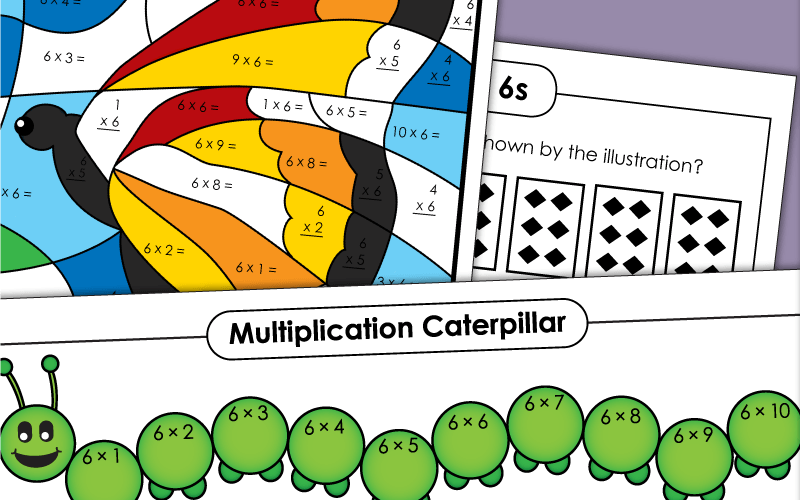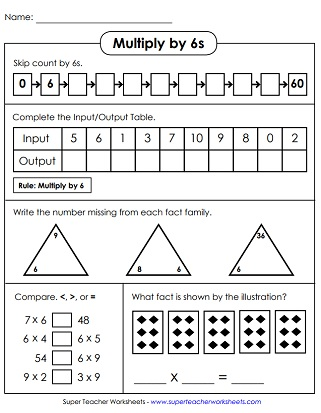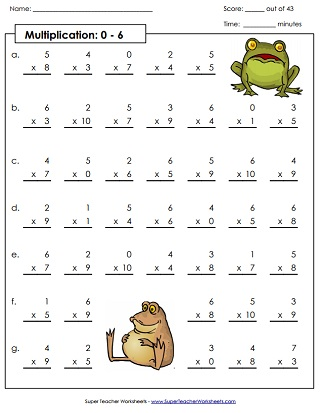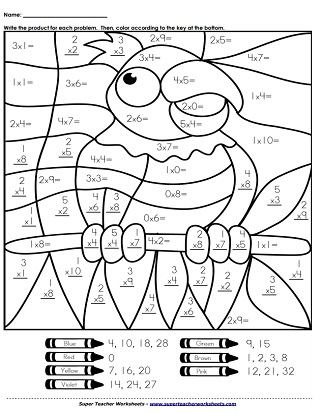# Multiplying by 6s

Basic worksheets for teaching kids to multiply by 6. Includes basic facts 0 through 6, as well as worksheets on 6s only, and skip counting by 6.## Multiplication by6s Only

After skip counting by 6s from 0 to 60, students will then complete an input-output table. After that, they'll write the missing factors in the fact family triangles.
Multiply by 6s. Write the answers in each space of the illustration. Then color according to the key at the bottom. What's the finished mystery picture? A butterfly!
These task cards can be used for peer practice, classroom scavenger hunts, exit slips, class games, and more.
Practice multiplication facts focusing on 5s and 6s with this printable cut-out activity.
In this printable math activity, students will multiply by 6s in order to fill out all the bubbles on the multiplication caterpillar.
Make an origami cootie catcher toy (also known as a "fortune teller"). Use it to learn basic facts with 6 as a factor.
Cut out the flash cards and use them to practice multiplication facts with 6 as a factor.
Print on card stock. Cut out and color the boat. Slide the multiplication strips through the boat. Slide the strip up and down to see basic facts. Read and answer each fact.

## Skip Count by 6s

Use your knowledge of skip counting to answer the logical and critical thinking questions.
Skip count by 6s using these number lines. Find the missing number to write into the boxes.
Starting at number 0, count by 6s and draw the line connecting the dots to form a shooting star.
Count by 6s up to 144. Write the answers on the bellies of the penguins.

## Sample Worksheet Images## All Facts0s through 6s

This printable drill worksheet has 43 multiplication problems, with factors of 0, 1, 2, 3, 4, 5 and 6. Facts through 10, up to 6x10.
This dog and cat-themed drill worksheet contains basic facts up to 6s. THis version includes 11s and 12s, up to 6x12.
This timed test will assess student knowledge of all facts, from 0x0 up to 6x12.
Roll two dice. Multiply the two numbers together. With standard dice, all rolls should be between 1x1 and 6x6.
Players take turns rolling a pair of dice. They multiply the numbers together. The player with the highest total wins the round. Play 18 rounds in all. With standard dice, the facts will go up to 6x6.
Solve the multiplication facts. Facts cover 0s, 1s, 2s, 3s, 4s, 5s, and 6s. Then color according to the key. Finished product is a parrot.
Multiplication Basic Facts: 0-10

Here you'll find lots more multiplication worksheets, games, and activities for all basic facts up to 10x10.

Multiplication/Division
Fact Families

This page has lots of worksheets with multiplication and division fact families.

Multiplication by 3s

Multiplication by 4s

These worksheets focus specifically on the 4s times tables.

Multiplication by 5s

Learning the 5s times tables only? Here's the page you're looking for.

Multiplication by 7s

On this page, you can download printables for practicing basic facts up to 7. There's a castle mystery picture, a dot-to-dot with 7s times tables, and more.

Multiplication by 8s

Practice multiplying by the number 8. Includes skip counting activities, mystery picture pages, a timed quiz, and more.

Multiplication by 9s

When you get to the 9s, check out this page. There's a 9s "trick" that you can teach your students. And, as always, mystery pictures, skip counting, and other worksheets.

My Account
Site Information